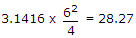# Electronics and Communication Engineering - Microprocessors

### Exercise :: Microprocessors - Section 3

21.

Zip floppy has a capacity of

 A. 50 MB B. 500 MB C. 25 MB D. 100 MB or 250 MB

Explanation:

Zip floppy has high memory 100 MB or 250 MB.

22.

Which of the following is not a valid character constant in C?

 A. 'A' B. '2' C. '=' D. 2

Explanation:

Character constant must be enclosed within single inverted commas.

23.

Consider the following program in Basic
`5 READ R 10 D = 2 * R 20 A = 3.1416 * D * D / 4 30 PRINT A`
Assuming that R = 3.0, the output A =

 A. 2.827 B. 28.27 C. 282.7 D. 2827

Explanation:.

24.

Consider the following statements about Java variables

1. Java variable must start with a letter or underscore or dollor sign.
3. After the first character the variable can have any combination of letters or numbers.
4. Capitalization of letters must be consistent.
Which of the above are correct?

 A. All B. 1, 2 and 3 only C. 2, 3, 4 only D. 1, 2 and 4 only

Explanation:

All these are correct for Java variables.

25.

A microprocessor is generally

 A. single chip SSI B. single chip MSI C. single chip LSI D. any of the above